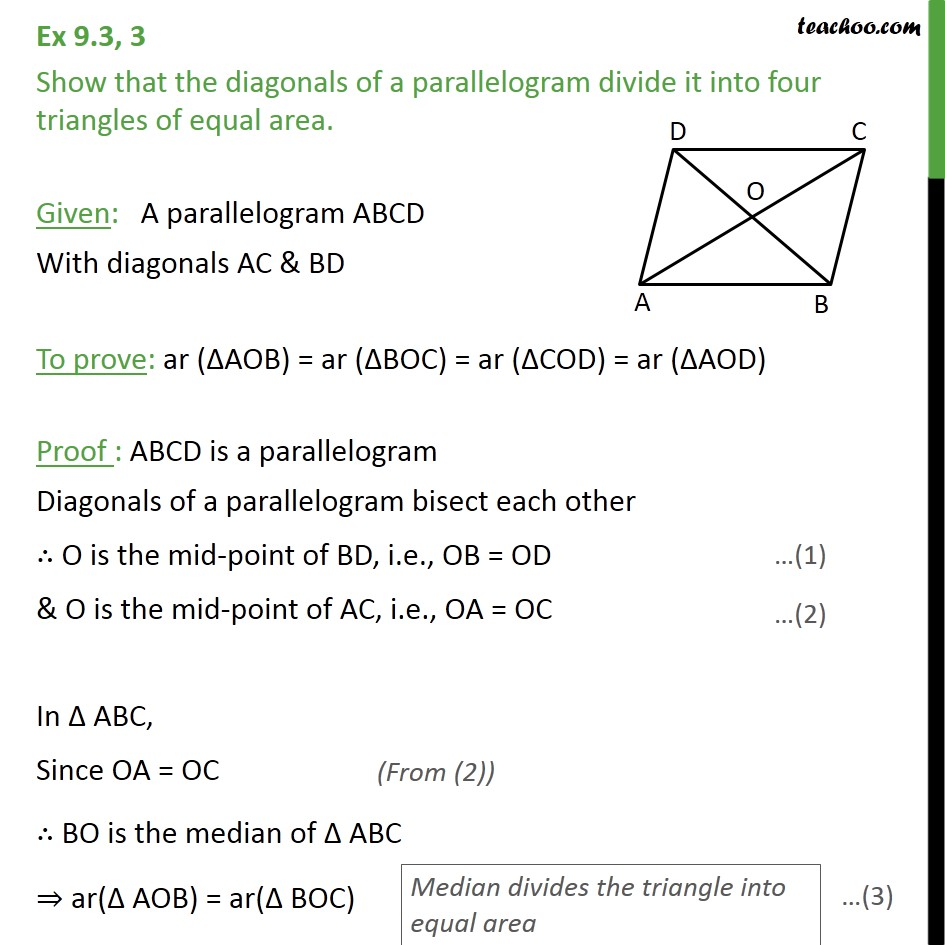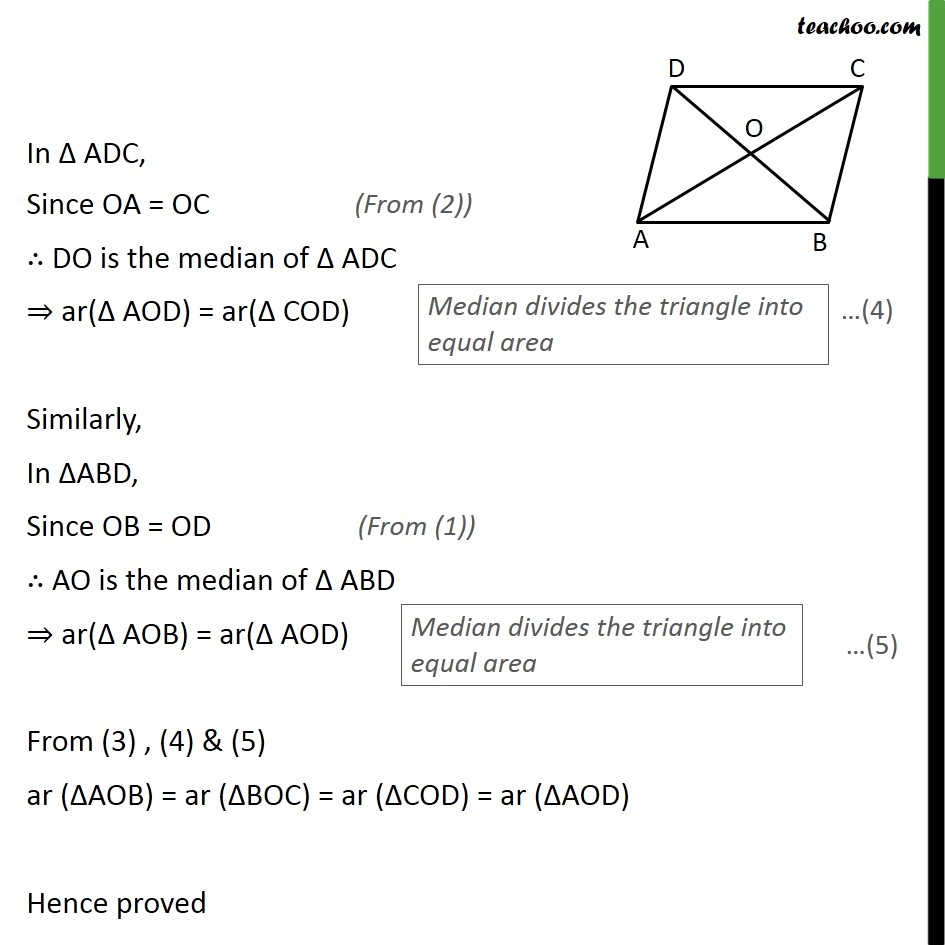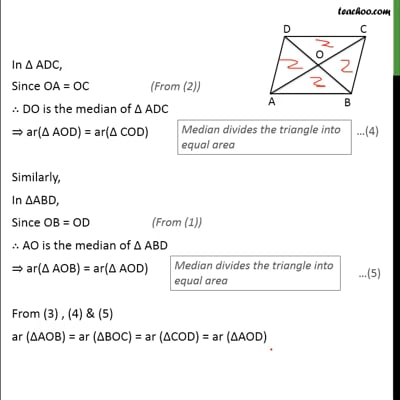Ex 9.3

Chapter 9 Class 9 - Areas of Parallelograms and Triangles [Deleted]
Serial order wiseThis video is only available for Teachoo black users

Since rectangle, square, rhombus are all parallelograms.

We can also say that

Diagonals of a rectangle divide it into 4 triangles of equal area

OR

Diagonals of a square divide it into 4 triangles of equal area

OR

Diagonals of a rhombus divide it into 4 triangles of equal area

Solve all your doubts with Teachoo Black (new monthly pack available now!)

### Transcript

Ex 9.3, 3 Show that the diagonals of a parallelogram divide it into four triangles of equal area. Given: A parallelogram ABCD With diagonals AC & BD To prove: ar (∆AOB) = ar (∆BOC) = ar (∆COD) = ar (∆AOD) Proof : ABCD is a parallelogram Diagonals of a parallelogram bisect each other ∴ O is the mid-point of BD, i.e., OB = OD & O is the mid-point of AC, i.e., OA = OC In ∆ ABC, Since OA = OC ∴ BO is the median of ∆ ABC ⇒ ar(∆ AOB) = ar(∆ BOC) In ∆ ADC, Since OA = OC ∴ DO is the median of ∆ ADC ⇒ ar(∆ AOD) = ar(∆ COD) Similarly, In ∆ABD, Since OB = OD ∴ AO is the median of ∆ ABD ⇒ ar(∆ AOB) = ar(∆ AOD) From (3) , (4) & (5) ar (∆AOB) = ar (∆BOC) = ar (∆COD) = ar (∆AOD) Hence proved